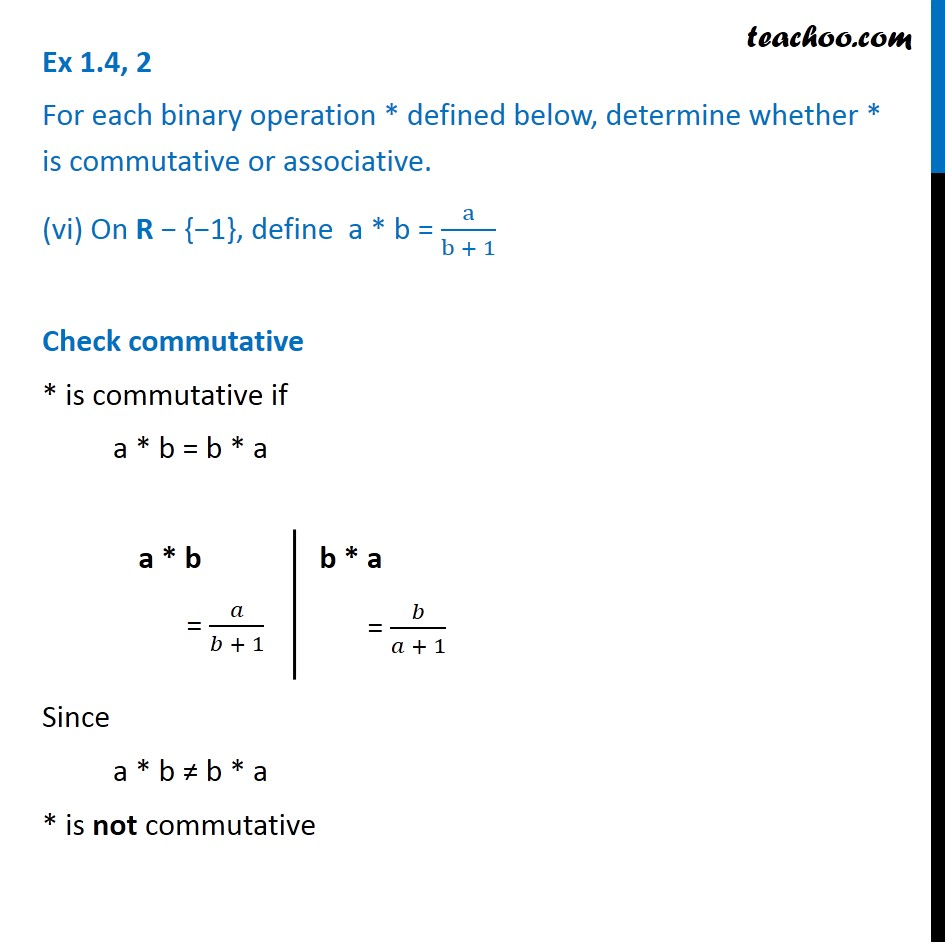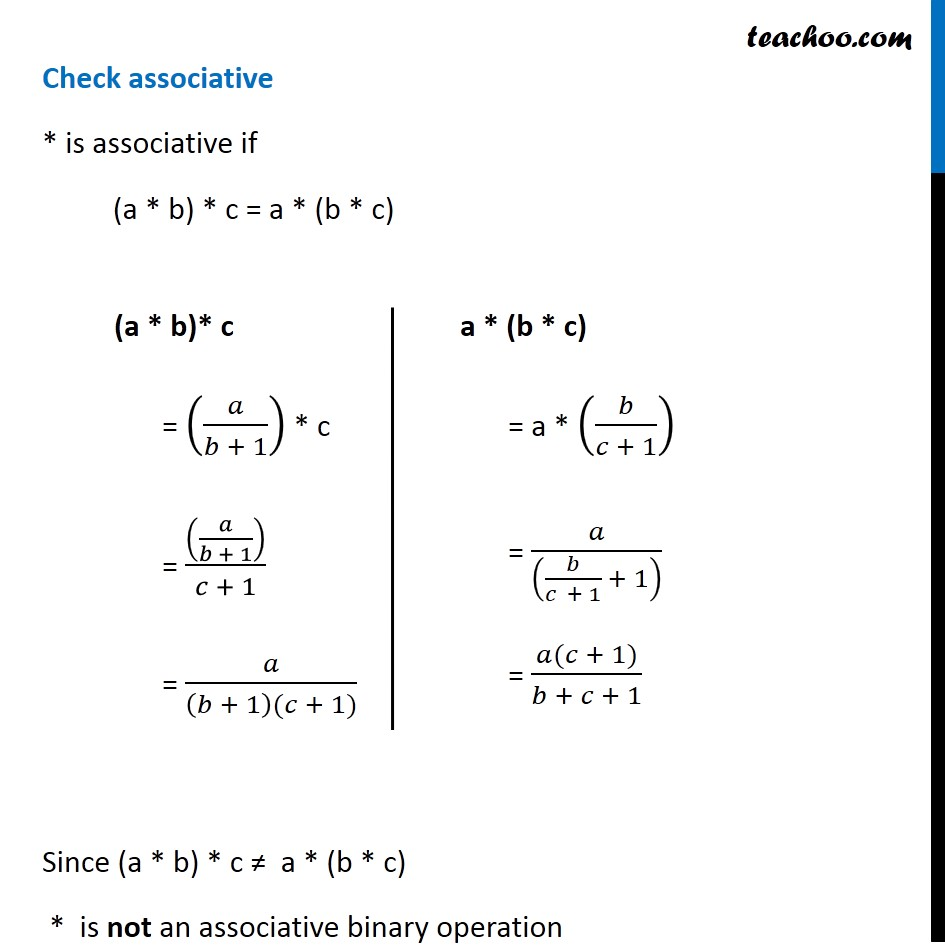Binary Operations

Chapter 1 Class 12 Relation and Functions
Serial order wiseLearn in your speed, with individual attention - Teachoo Maths 1-on-1 Class

### Transcript

Ex 1.4, 2 For each binary operation * defined below, determine whether * is commutative or associative. (vi) On R − {−1}, define a * b = a/(b + 1) Check commutative * is commutative if a * b = b * a Since a * b ≠ b * a * is not commutative a * b = 𝑎/(𝑏 + 1) b * a = 𝑏/(𝑎 + 1) Check associative * is associative if (a * b) * c = a * (b * c) Since (a * b) * c ≠ a * (b * c) * is not an associative binary operation (a * b)* c = (𝑎/(𝑏 + 1)) * c = ((𝑎/(𝑏 + 1)))/(𝑐 + 1) = 𝑎/((𝑏 + 1)(𝑐 + 1)) a * (b * c) = a * (𝑏/(𝑐 + 1)) = 𝑎/((𝑏/(𝑐 + 1) + 1) ) = (𝑎(𝑐 + 1))/(𝑏 + 𝑐 + 1)